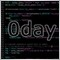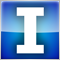# How to compare doubles ?117

To compare 1.36 with 1.36 i need to use

if(CompareDoubles(x , y)){

// the same

}

But how to compare like this ?

if ( 1.36 >= 1.36 ){

?

Does this bug with compare doubles are only with == ? or with > < too ?

thanks

the only correct way in my mind is:

if(CompareDoubles(x , y) || x > y){ // correct ?

Or the more simply method is this:

if(NormalizeDouble(x, 5) >= NormalizeDouble(y, 5)){

// i think this method is the correct way to compare dubles, yes ?

}23647

Everything depends on the type of comparison you want to ask:

x <y

x> y

x <= y

x> = y

x == y

x! = y

``` if (CompareDoubles(( double )x , ( double )y))
{
// the same
}

bool CompareDoubles( double X, double Y)
{
if ( X > Y) return ( true );

return ( false );
}```38097

Can price != price ? - MQL4 forum
• www.mql5.com
Can price != price ? - MQL4 forum